# Number Line

Did you know that the number line was invented by Ancient Egyptians with a rope to measure lengths? This was done 2500 years ago, and with it, they were able to carry out number line addition and number line subtraction.

Over millennia, philosophers, scientists, and mathematicians discovered interactive number lines, negative number lines, number line integers, and with their help, we will try and solve some number line examples.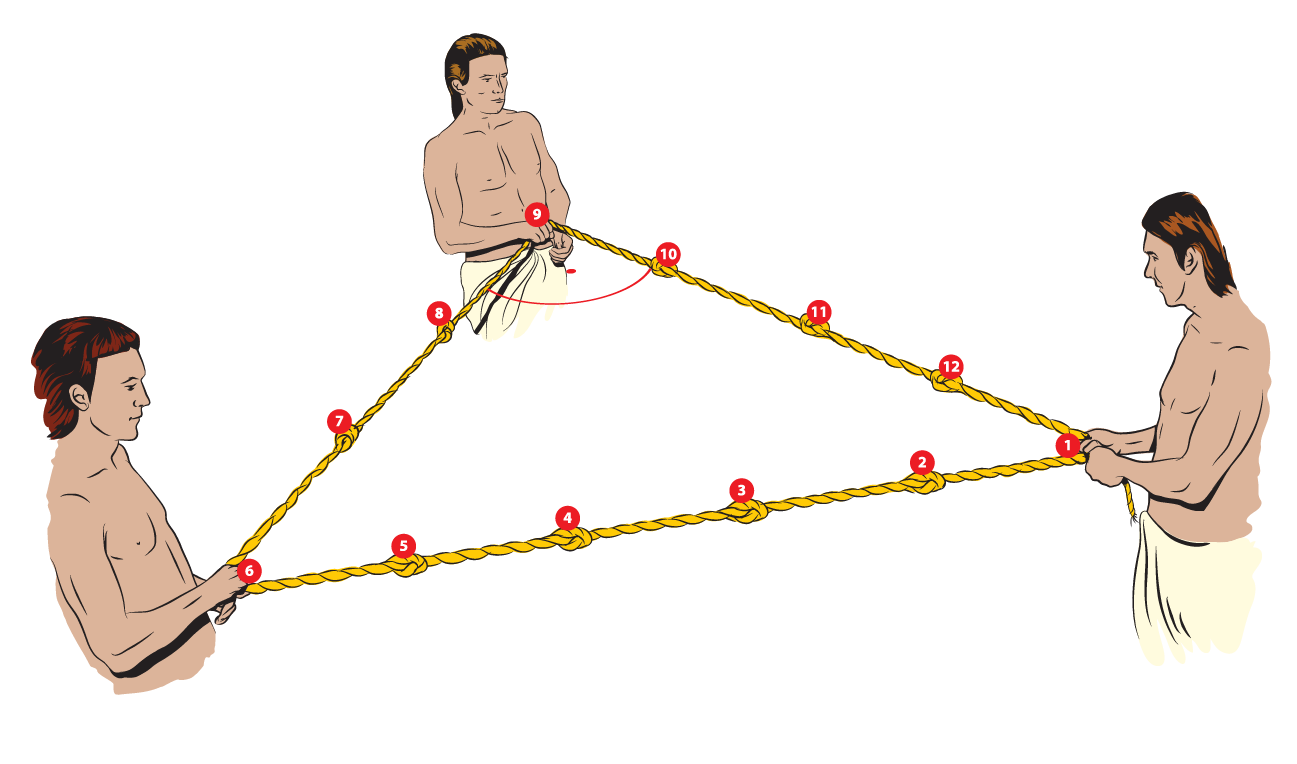You can check out the interactive simulations to know more about this lesson and try your hand at solving a few interesting practice questions at the end of the page.

## Lesson Plan

 1 What Is a Number Line? 2 Solved Examples on Number Lines 3 Interactive Questions on Number Lines

## What Is a Number Line?

A number line is a visual representation of numbers on a straight line.

This line is used to compare numbers that are placed at equal intervals on an infinite line that extends on both sides, horizontally.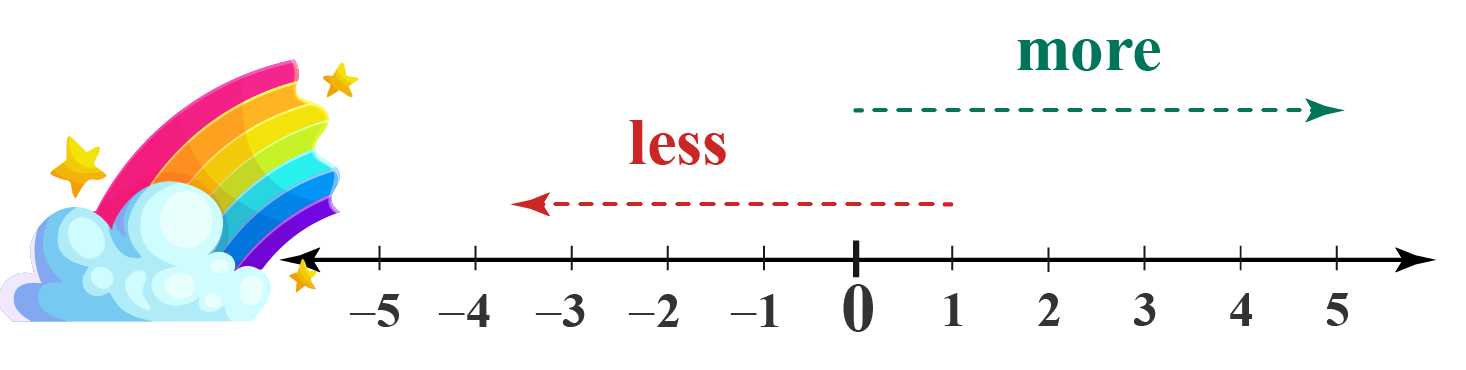## Integers on a Number Line

On a number line, integers are marked on equal intervals.

### Positive Number Line and Negative Number Line

If we observe the given number line, we find that the origin is $$0$$ (zero), which stands in the middle.

To the right, there are positive numbers and to the left, there are negative numbers.

Hence, this number line represents integers which include positive and negative numbers.

These extend endlessly in both directions and that is why the arrows are marked at both ends to show that the line is infinite.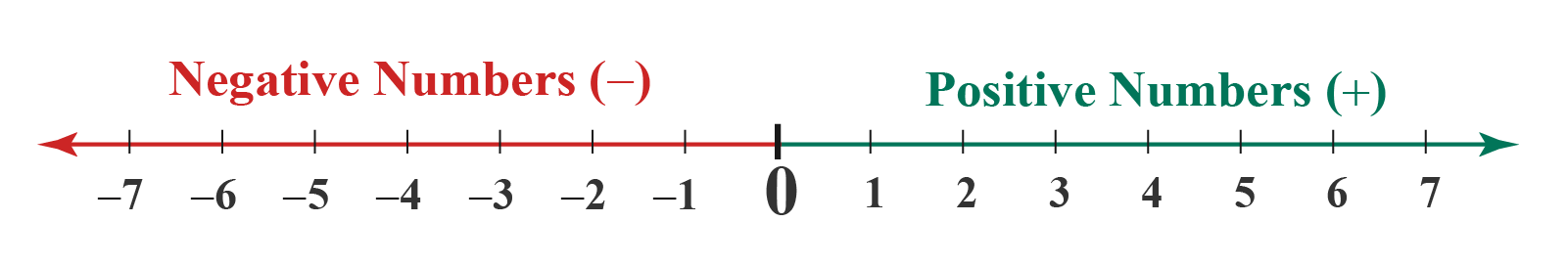### The numbers increase and decrease as we change directions

Any number to the left is always less than the number to its right.

For example:

$$2$$ is less than $$4$$

-$$3$$ is less than -$$1$$

Similarly, any number to the right is always more than the number to its left.

For example:

$$5$$ is greater than $$3$$

-$$1$$ is greater than -$$3$$

## Use of Number Lines

In math, number line has applications for the understanding of number operations, which are considered as basics or foundation of math.

### Number Line Addition

Let's assume that you have $$2$$ flowers. Your friend gave you $$3$$ more.

Find the number of flowers you have now.

How will you solve it using a number line?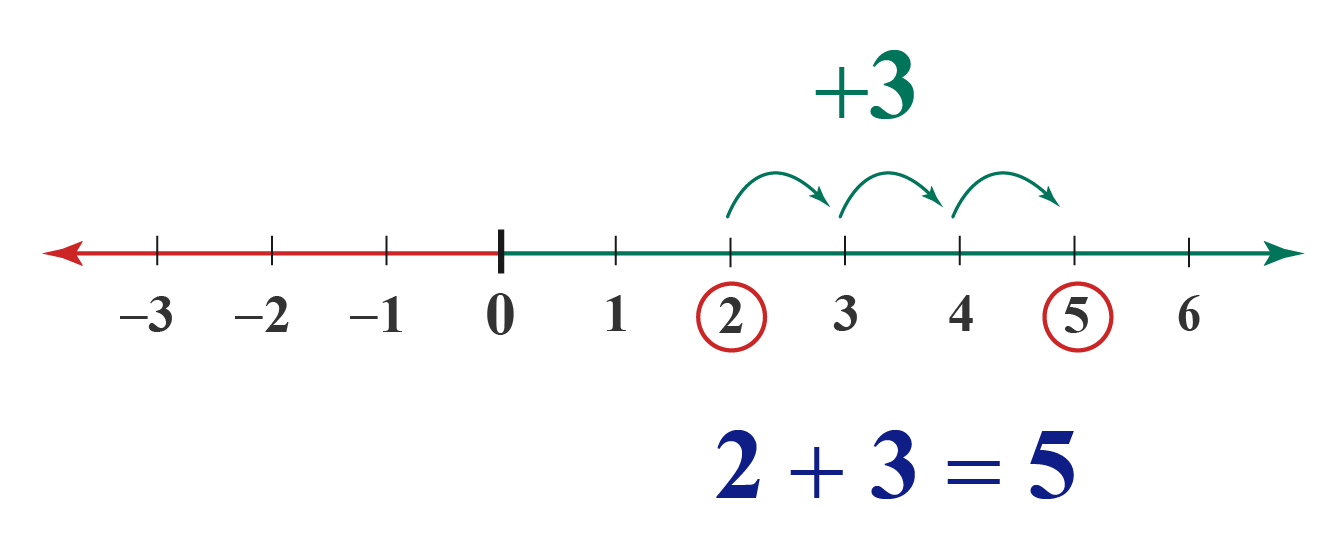We know that the numbers increase as we go to the right.

Starting from $$2$$, take $$3$$ steps to the right to get the answer!

Now you have $$5$$ flowers!

### Number Line Subtraction

Let's say, you have $$7$$ oranges and you give $$3$$ to your friend.

Find the number of oranges you have now.

How will you solve this using a number line?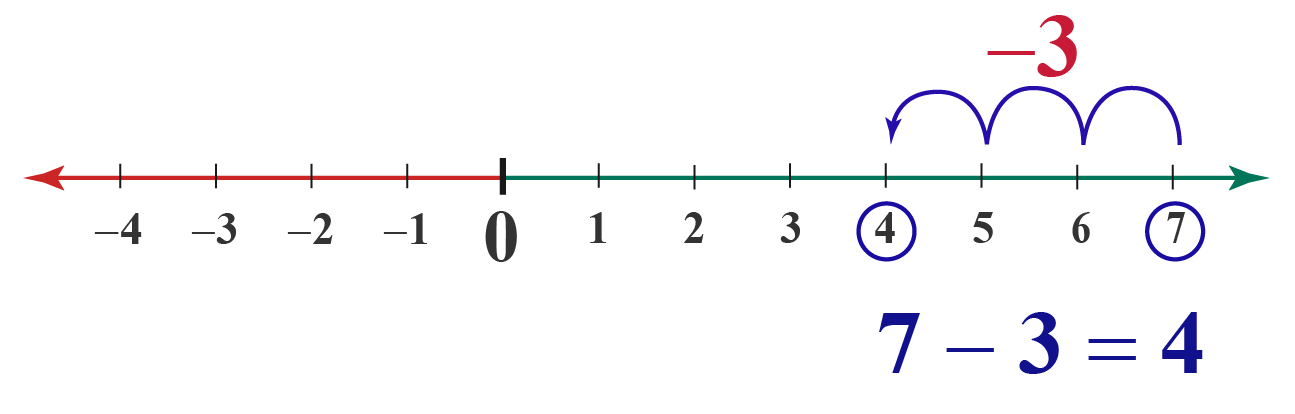We know that the numbers decrease as we go to the left.

Starting from $$7$$, take $$3$$ steps to the left to get the answer!

Now you have $$4$$ oranges left!

### Solving Negative Numbers

Number lines help us understand negative numbers and solve questions like the following.

What is $$3$$ more than -$$1$$?

Solution:

Let us represent this on a number line.

This helps us understand the position of -$$1$$, and where it reaches after moving $$3$$ steps to its right.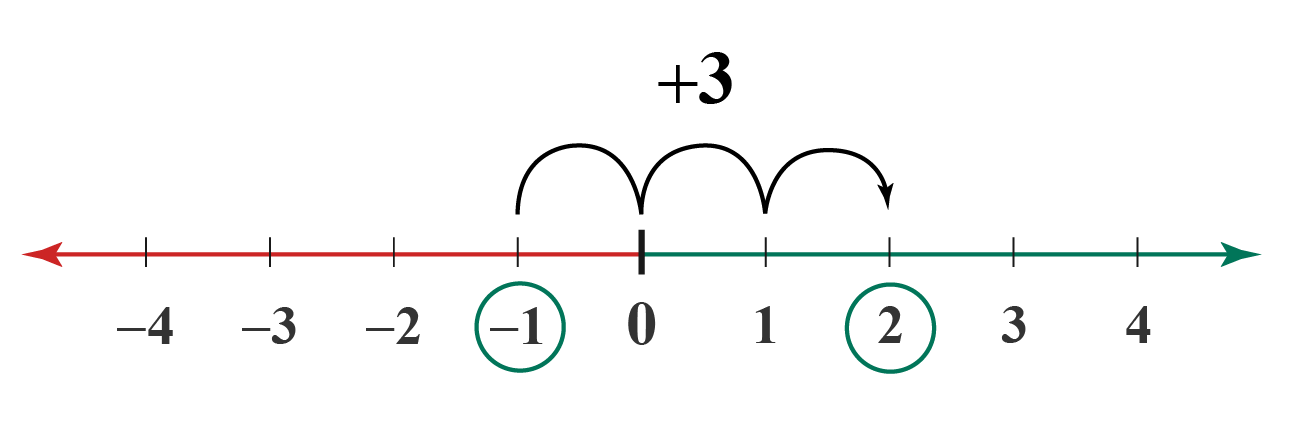We move $$3$$ steps to the right of -$$1$$ (A Number to the right is always greater than the number to the left).

Thus, the answer is $$2$$

## Interactive Number Lines

Try this interactive number line to observe number line addition and subtraction.

Reset, Enter the answer and Check!

## Solved Examples

Here are a few number line examples. Let's have a look!

## Interactive Questions

Try these Questions based on the Number Line in the worksheet given below.

Select/Type your answer and click the "Check Answer" button to see the result.

## Let's Summarize

We hope you enjoyed learning about the number line with the simulations and practice questions. Now you will be able to easily solve problems on number lines, number line addition, interactive number lines, negative number lines, number line integers, number line examples, and number line with negatives.

## About Cuemath

At Cuemath, our team of math experts is dedicated to making learning fun for our favorite readers, the students!

Through an interactive and engaging learning-teaching-learning approach, the teachers explore all angles of a topic.

Be it worksheets, online classes, doubt sessions, or any other form of relation, it’s the logical thinking and smart learning approach that we at Cuemath believe in.

## 1. How do you write a number line?

A number line usually has its origin with the number $$0$$ (zero) located in the middle.

The positive numbers are marked to its right, whereas negative numbers are plotted to its left, in an order where each number is placed at equal intervals.

## 2. What is the definition of the number line?

A number line is a visual representation of numbers on a straight line.

## 3. What is a number line model?

A number line model is a representation of numbers plotted on an infinite line which extends at both ends.

More Important Topics
Numbers
Algebra
Geometry
Measurement
Money
Data
Trigonometry
Calculus